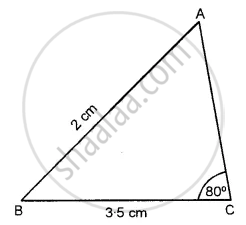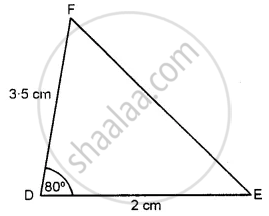Advertisement Remove all ads

# State, Whether the Pairs of Triangles Given in the Following Figures Are Congruent Or Not: δ Abc in Which Ab = 2 Cm, Bc = 3.5 Cm and ∠C = 80° and δ Def in Which De = 2 Cm, Df = 3.5 Cm and ∠D = 80°. - Mathematics

Diagram

State, whether the pairs of triangles given in the following figures are congruent or not:

Δ ABC in which AB = 2 cm, BC = 3.5 cm and ∠C = 80° and Δ DEF in which DE = 2 cm, DF = 3.5 cm and ∠D = 80°.

Advertisement Remove all ads

#### Solution

In Δ ABC, AB = 2 cm, BC = 3.5 cm and ∠C = 80° and in ∆ DEF, DE = 2 cm, DF = 3.5 cm and ∠D = 80°From the figure, we see that two corresponding sides are equal but their included angles are not equal.
Hence, these are not congruent triangles

Is there an error in this question or solution?
Advertisement Remove all ads

#### APPEARS IN

Selina Concise Mathematics Class 7 ICSE
Chapter 19 Congruency: Congruent Triangles
Exercise 19 | Q 1.7
Advertisement Remove all ads
Advertisement Remove all ads
Share
Notifications

View all notifications

Forgot password?
Course